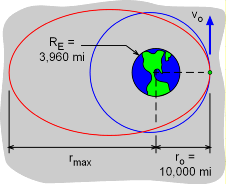Ch 2. Particle Force and Acceleration Multimedia Engineering Dynamics Rect.Coord. Normal/Tang. Coord. PolarCoord. Orbital Mechanics Computational Mechanics
 Chapter - Particle - 1. General Motion 2. Force & Accel. 3. Energy 4. Momentum - Rigid Body - 5. General Motion 6. Force & Accel. 7. Energy 8. Momentum 9. 3-D Motion 10. Vibrations Appendix Basic Math Units Basic Equations Sections Search eBooks Dynamics Fluids Math Mechanics Statics Thermodynamics Author(s): Kurt Gramoll ©Kurt GramollDYNAMICS - CASE STUDY IntroductionProblem Diagram A satellite is carried by the Space Shuttle to a height of 10,000 miles from the center of the earth and released. The astronauts on the Space Shuttle need to know what the initial velocity of the satellite should be for it to attain the correct orbit. What is known: The radius of the earth is RE = 3,960 miles. The initial radius of the satellite from the center of the earth is ro = 10,000 miles, which is 6,040 miles from the surface of the earth. The initial velocity of the satellite will be parallel to the tangent to the earth's surface. Question What initial velocity should the satellite have if the orbit is to be circular? What is the minimum velocity required for the satellite to escape the earth's gravitational pull? If the initial velocity is 20,000 ft/s, what is the maximum radius of the orbit? Approach Use polar coordinates and the equation that describes the free-flight trajectory of an orbiting satellite. Determine the relationship between the free-flight trajectory and the eccentricity of a conic section.

Practice Homework and Test problems now available in the 'Eng Dynamics' mobile app
Includes over 400 problems with complete detailed solutions.
Available now at the Google Play Store and Apple App Store.